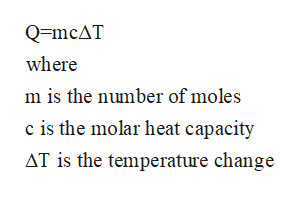# A chemist carefully measures the amount of heat needed to raise the temperature of a 2.00kg sample of C2H2Cl2 from 6.3°C to 16.5°C. The experiment shows that ×2.25104J of heat are needed. What can the chemist report for the molar heat capacity of C2H2Cl2? Be sure your answer has the correct number of significant digits.

Question

A chemist carefully measures the amount of heat needed to raise the temperature of a 2.00kg sample of C2H2Cl2 from 6.3°C to 16.5°C. The experiment shows that ×2.25104J of heat are needed. What can the chemist report for the molar heat capacity of C2H2Cl2? Be sure your answer has the correct number of significant digits.

check_circleExpert Solution
Step 1

Given data

Mass of C2H2Cl2 = 2.0kg

Total heat = 2.25x104 J

Temperature difference = (16.5 - 6.3)°C

Step 2

The equation for heat neededhelp_outlineImage TranscriptioncloseQ=mcAT where m is the number of moles c is the molar heat capacity AT is the temperature change fullscreen
Step 3

No of moles of the substance provid...

### Want to see the full answer?

See Solution

#### Want to see this answer and more?

Solutions are written by subject experts who are available 24/7. Questions are typically answered within 1 hour*

See Solution
*Response times may vary by subject and question
Tagged in

### Chemical Thermodynamics